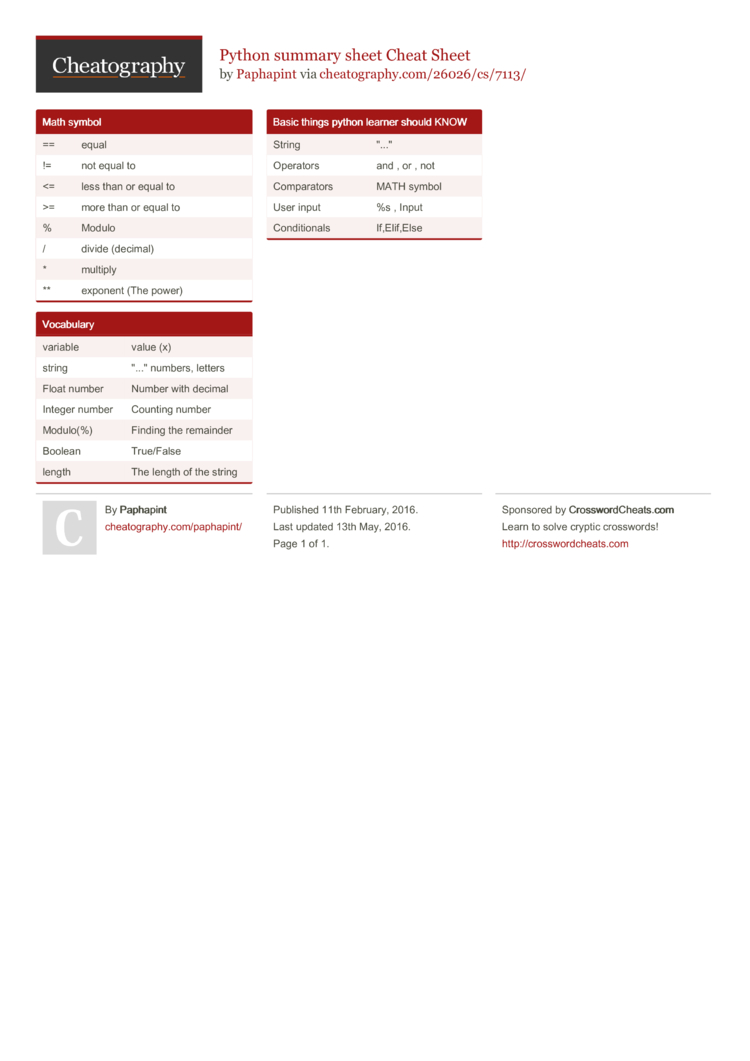# Python summary sheet Cheat Sheet by Paphapint

### Math symbol

 == equal != not equal to <= less than or equal to >= more than or equal to % Modulo / divide (decimal) * multiply ** exponent (The power)

### Vocabulary

 variable value (x) string "..."­ numbers, letters Float number Number with decimal Integer number Counting number Modulo(%) Finding the remainder Boolean True/False length The length of the string

### Basic things python learner should KNOW

 String "..."­ Operators and , or , not Compar­ators MATH symbol User input %s , Input Condit­ionals If,Eli­f,Else1 Page
//media.cheatography.com/storage/thumb/paphapint_python-summary-sheet.750.jpg

PDF (recommended)